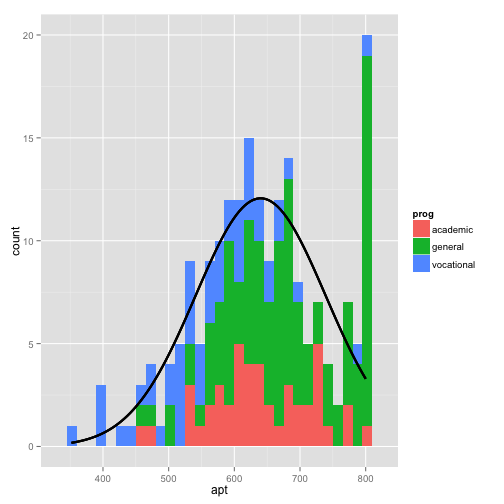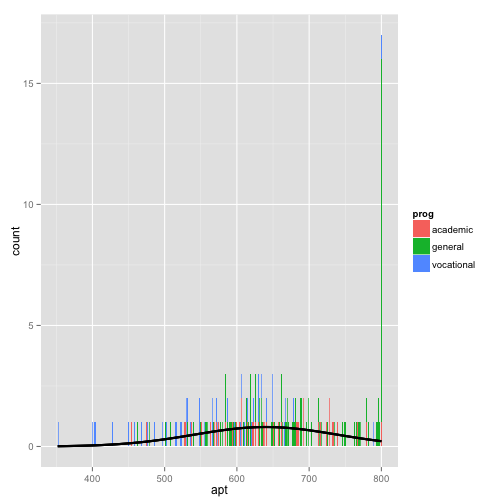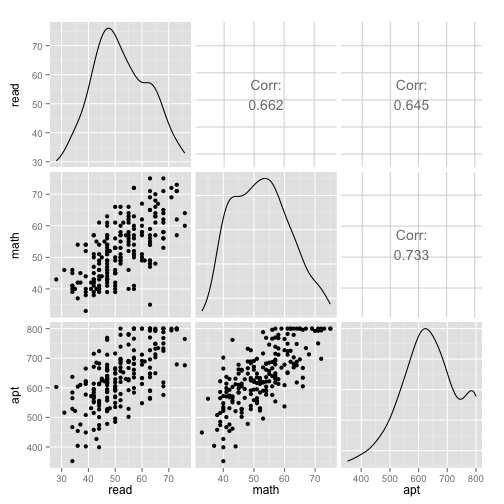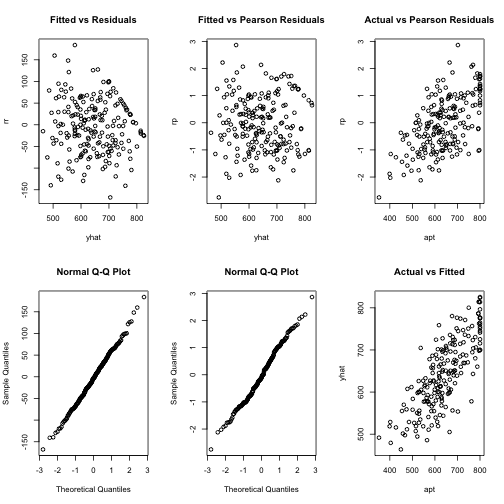# Tobit regression

The tobit model, also called a censored regression model, is designed to estimate linear relationships between variables when there is either left- or right-censoring in the dependent variable (also known as censoring from below and above, respectively). Censoring from above takes place when cases with a value at or above some threshold, all take on the value of that threshold, so that the true value might be equal to the threshold, but it might also be higher. In the case of censoring from below, values those that fall at or below some threshold are censored.

```library(VGAM)
```
```## Loading required package: stats4
##
## Attaching package: 'VGAM'
##
## The following objects are masked from 'package:boot':
##
##     logit, simplex
##
## The following object is masked from 'package:coda':
##
##     nvar
```
```library(GGally)
library(ggplot2)
```

## Examples of tobit analysis

• Example 1. In the 1980s there was a federal law restricting speedometer readings to no more than 85 mph. So if you wanted to try and predict a vehicle's top-speed from a combination of horse-power and engine size, you would get a reading no higher than 85, regardless of how fast the vehicle was really traveling. This is a classic case of right-censoring (censoring from above) of the data. The only thing we are certain of is that those vehicles were traveling at least 85 mph.

• Example 2. A research project is studying the level of lead in home drinking water as a function of the age of a house and family income. The water testing kit cannot detect lead concentrations below 5 parts per billion (ppb). The EPA considers levels above 15 ppb to be dangerous. These data are an example of left-censoring (censoring from below).

• Example 3. Consider the situation in which we have a measure of academic aptitude (scaled 200-800) which we want to model using reading and math test scores, as well as, the type of program the student is enrolled in (academic, general, or vocational). The problem here is that students who answer all questions on the academic aptitude test correctly receive a score of 800, even though it is likely that these students are not "truly" equal in aptitude. The same is true of students who answer all of the questions incorrectly. All such students would have a score of 200, although they may not all be of equal aptitude.

# Academic aptitude data

For our data analysis below, we are going to expand on Example 3 from above. We have generated hypothetical data, which can be obtained from our website from within R. Note that R requires forward slashes, not back slashes when specifying a file location even if the file is on your hard drive.

```dat <- read.csv("http://www.karlin.mff.cuni.cz/~pesta/prednasky/NMFM404/Data/tobit.csv")
```

The dataset contains 200 observations. The academic aptitude variable is `apt`, the reading and math test scores are `read` and `math` respectively. The variable `prog` is the type of program the student is in, it is a categorical (nominal) variable that takes on three values, academic (`prog` = 1), general (`prog` = 2), and vocational (`prog` = 3). The variable `id` is an identification variable.

Now let's look at the data descriptively. Note that in this dataset, the lowest value of apt is 352. That is, no students received a score of 200 (the lowest score possible), meaning that even though censoring from below was possible, it does not occur in the dataset.

```summary(dat)
```
```##        id              read            math               prog
##  Min.   :  1.00   Min.   :28.00   Min.   :33.00   academic  : 45
##  1st Qu.: 50.75   1st Qu.:44.00   1st Qu.:45.00   general   :105
##  Median :100.50   Median :50.00   Median :52.00   vocational: 50
##  Mean   :100.50   Mean   :52.23   Mean   :52.65
##  3rd Qu.:150.25   3rd Qu.:60.00   3rd Qu.:59.00
##  Max.   :200.00   Max.   :76.00   Max.   :75.00
##       apt
##  Min.   :352.0
##  1st Qu.:575.5
##  Median :633.0
##  Mean   :640.0
##  3rd Qu.:705.2
##  Max.   :800.0
```

```# function that gives the density of normal distribution
# for given mean and sd, scaled to be on a count metric
# for the histogram: count = density * sample size * bin width
f <- function(x, var, bw = 15) {
dnorm(x, mean = mean(var), sd(var)) * length(var)  * bw
}

# setup base plot
p <- ggplot(dat, aes(x = apt, fill=prog))

# histogram, coloured by proportion in different programs
# with a normal distribution overlayed
p + stat_bin(binwidth=15) +
stat_function(fun = f, size = 1,
args = list(var = dat\$apt))
```Looking at the above histogram, we can see the censoring in the values of `apt`, that is, there are far more cases with scores of 750 to 800 than one would expect looking at the rest of the distribution. Below is an alternative histogram that further highlights the excess of cases where `apt`=800. In the histogram below, the `breaks` option produces a histogram where each unique value of `apt` has its own bar (by setting breaks equal to a vector containing values from the minimum of `apt` to the maximum of `apt`). Because `apt` is continuous, most values of `apt` are unique in the dataset, although close to the center of the distribution there are a few values of `apt` that have two or three cases. The spike on the far right of the histogram is the bar for cases where `apt`=800, the height of this bar relative to all the others clearly shows the excess number of cases with this value.

```p + stat_bin(binwidth = 1) + stat_function(fun = f, size = 1, args = list(var = dat\$apt,
bw = 1))
```Next we'll explore the bivariate relationships in our dataset.

```cor(dat[, c("read", "math", "apt")])
```
```##           read      math       apt
## read 1.0000000 0.6622801 0.6451215
## math 0.6622801 1.0000000 0.7332702
## apt  0.6451215 0.7332702 1.0000000
```

```# plot matrix
ggpairs(dat[, c("read", "math", "apt")])
```In the first row of the scatterplot matrix shown above, we see the scatterplots showing the relationship between `read` and `apt`, as well as `math` and `apt`. Note the collection of cases at the top these two scatterplots, this is due to the censoring in the distribution of `apt`.

## Analysis methods you might consider

Below is a list of some analysis methods you may have encountered. Some of the methods listed are quite reasonable while others have either fallen out of favor or have limitations.

• Tobit regression - the focus of this page.
• OLS Regression - You could analyze these data using OLS regression. OLS regression will treat the 800 as actual values and not as the lower limit of the top academic aptitude. A limitation of this approach is that when the variable is censored, OLS provides inconsistent estimates of the parameters, meaning that the coefficients from the analysis will not necessarily approach the "true" population parameters as the sample size increases. See Long (1997, chapter 7) for a more detailed discussion of problems of using OLS regression with censored data.
• Truncated Regression - There is sometimes confusion about the difference between truncated data and censored data. With censored variables, all of the observations are in the dataset, but we don't know the "true" values of some of them. With truncation some of the observations are not included in the analysis because of the value of the variable. When a variable is censored, regression models for truncated data provide inconsistent estimates of the parameters. See Long (1997, chapter 7) for a more detailed discussion of problems of using regression models for truncated data to analyze censored data.

# Tobit model

Below we run the tobit model, using the `vglm` function of the `VGAM` package.

```summary(m <- vglm(apt ~ read + math + prog, tobit(Upper = 800), data = dat))
```
```##
## Call:
## vglm(formula = apt ~ read + math + prog, family = tobit(Upper = 800),
##     data = dat)
##
## Pearson residuals:
##             Min      1Q  Median     3Q   Max
## mu       -2.752 -0.7879 -0.1124 0.7307 2.864
## loge(sd) -1.149 -0.6228 -0.3336 0.2296 4.439
##
## Coefficients:
##                 Estimate Std. Error z value Pr(>|z|)
## (Intercept):1  209.56592   32.43546   6.461 1.04e-10 ***
## (Intercept):2    4.18474    0.05229  80.036  < 2e-16 ***
## read             2.69794    0.61780   4.367 1.26e-05 ***
## math             5.91449    0.70296   8.414  < 2e-16 ***
## proggeneral    -12.71476   12.38655  -1.026 0.304657
## progvocational -46.14388   13.64862  -3.381 0.000723 ***
## ---
## Signif. codes:  0 '***' 0.001 '**' 0.01 '*' 0.05 '.' 0.1 ' ' 1
##
## Number of linear predictors:  2
##
## Names of linear predictors: mu, loge(sd)
##
## Dispersion Parameter for tobit family:   1
##
## Log-likelihood: -1041.063 on 394 degrees of freedom
##
## Number of iterations: 5
```

• In the output above, the first thing we see is the call, this is R reminding us what the model we ran was, what options we specified, etc.
• The table labeled coefficients gives the coefficients, their standard errors, and the z-statistic. No p-values are included in the summary table, but we show how to calculate them below. Tobit regression coefficients are interpreted in the similar manner to OLS regression coefficients; however, the linear effect is on the uncensored latent variable, not the observed outcome.
• For a one unit increase in `read`, there is a 2.6981 point increase in the predicted value of `apt`.
• A one unit increase in `math` is associated with a 5.9146 unit increase in the predicted value of `apt`.
• The terms for `prog` have a slightly different interpretation. The predicted value of `apt` is -46.1419 points lower for students in a vocational program than for students in an academic program.
• The coefficient labeled "(Intercept):1" is the intercept or constant for the model.
• The coefficient labeled "(Intercept):2" is an ancillary statistic. If we exponentiate this value, we get a statistic that is analogous to the square root of the residual variance in OLS regression. The value of 65.6773 can compared to the standard deviation of academic aptitude which was 99.21, a substantial reduction.
• The final log likelihood, -1041.0629, is shown toward the bottom of the output, it can be used in comparisons of nested models.
• Below we calculate the p-values for each of the coefficients in the model. We calculate the p-value for each coefficient using the z values and then display in a table with the coefficients. The coefficients for read, math, and prog = 3 (vocational) are statistically significant.

Below we calculate the p-values for each of the coefficients in the model. We calculate the p-value for each coefficient using the z values and then display in a table with the coefficients. The coefficients for `read`, `math`, and `prog` = 3 (vocational) are statistically significant.

```ctable <- coef(summary(m))
pvals <- 2 * pt(abs(ctable[, "z value"]), df.residual(m), lower.tail = FALSE)
cbind(ctable, pvals)
```
```##                  Estimate  Std. Error   z value     Pr(>|z|)         pvals
## (Intercept):1  209.565918 32.43546321  6.461012 1.040051e-10  3.072320e-10
## (Intercept):2    4.184745  0.05228575 80.036054 0.000000e+00 8.476290e-246
## read             2.697939  0.61780152  4.366999 1.259653e-05  1.612213e-05
## math             5.914487  0.70295676  8.413727 3.971836e-17  7.407558e-16
## proggeneral    -12.714759 12.38654725 -1.026497 3.046572e-01  3.052870e-01
## progvocational -46.143880 13.64861577 -3.380847 7.226280e-04  7.948536e-04
```

We can test the significant of program type overall by fitting a model without program in it and using a likelihood ratio test.

```m2 <- vglm(apt ~ read + math, tobit(Upper = 800), data = dat)

(p <- pchisq(2 * (logLik(m) - logLik(m2)), df = 2, lower.tail = FALSE))
```
```##  0.003155176
```

The LRT with two degrees of freedom is associated with a p-value of 0.0032, indicating that the overall effect of `prog` is statistically significant.

Below we calculate the upper and lower 95% confidence intervals for the coefficients.

```b <- coef(m)
se <- sqrt(diag(vcov(m)))

cbind(LL = b - qnorm(0.975) * se, UL = b + qnorm(0.975) * se)
```
```##                        LL         UL
## (Intercept):1  145.993578 273.138257
## (Intercept):2    4.082267   4.287223
## read             1.487070   3.908807
## math             4.536717   7.292256
## proggeneral    -36.991945  11.562428
## progvocational -72.894676 -19.393085
```

We may also wish to examine how well our model fits the data. One way to start is with plots of the residuals to assess their absolute as well as relative (pearson) values and assumptions such as normality and homogeneity of variance.

```dat\$yhat <- fitted(m)[,1]
dat\$rr <- resid(m, type = "response")
dat\$rp <- resid(m, type = "pearson")[,1]

par(mfcol = c(2, 3))

with(dat, {
plot(yhat, rr, main = "Fitted vs Residuals")
qqnorm(rr)
plot(yhat, rp, main = "Fitted vs Pearson Residuals")
qqnorm(rp)
plot(apt, rp, main = "Actual vs Pearson Residuals")
plot(apt, yhat, main = "Actual vs Fitted")
})
```The graph in the bottom right was the predicted, or fitted, values plotted against the actual. This can be particularly useful when comparing competing models. We can calculate the correlation between these two as well as the squared correlation, to get a sense of how accurate our model predicts the data and how much of the variance in the outcome is accounted for by the model.

```# correlation
(r <- with(dat, cor(yhat, apt)))
```
```##  0.782471
```
```# variance accounted for
r^2
```
```##  0.6122608
```

The correlation between the predicted and observed values of `apt` is 0.7825. If we square this value, we get the multiple squared correlation, this indicates predicted values share 61.23% of their variance with `apt`

## References

• UCLA: IDRE (Institute for Digital Research and Education). Data Analysis Examples. from http://www.ats.ucla.edu/stat/dae/ (accessed January 31, 2014)
• Long, J. S. (1997). Regression Models for Categorical and Limited Dependent Variables. Thousand Oaks, CA: Sage Publications.
• Tobin, J. 1958. Estimation of relationships for limited dependent variables. Econometrica 26: 24-36.